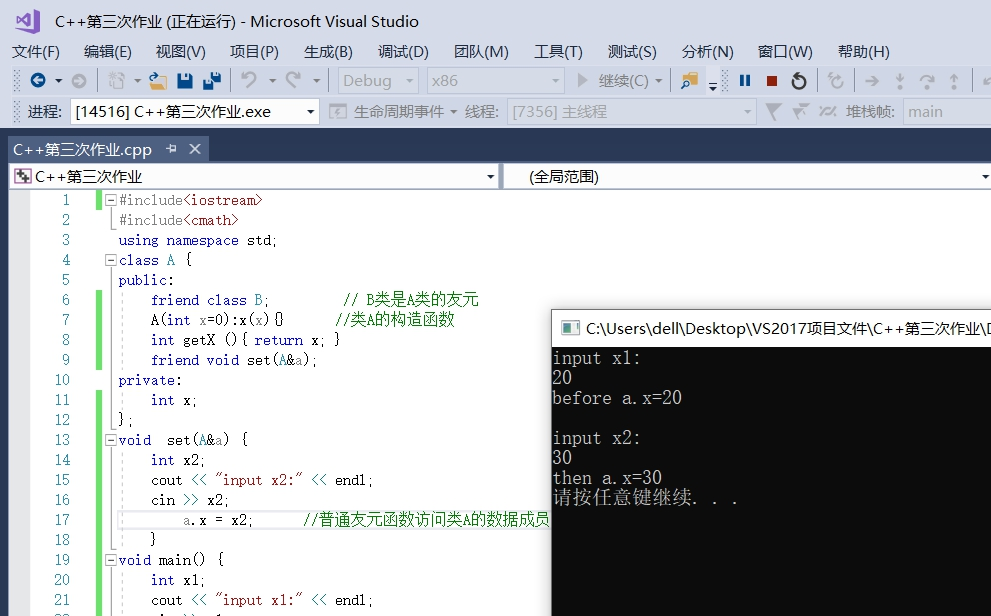# 类的友元

## 一、友元函数

friend + 函数声明


### 1.通过友元函数为普通函数的函数对类的数据成员进行访问

#include<iostream>
#include<cmath>
using namespace std;
class A {
public:
friend class B;         // B类是A类的友元
A(int x=0):x(x){}      //类A的构造函数
int getX (){ return x; }
friend void set(A&a);  //
private:
int x;
};
void  set(A&a) {
int x2;
cout << "input x2:" << endl;
cin >> x2;
a.x = x2;      //普通友元函数访问类A的数据成员
}
void main() {
int x1;
cout << "input x1:" << endl;
cin >> x1;
A a(x1);
cout <<"before a.x="<< a.getX() << endl<<endl;
set(a);
cout <<"then a.x="<< a.getX() << endl;
system("pause");

}##二、友元类 同友元函数一样，一个类可以将另一个类声明为友元类。若A类为B类的友元类，则A类的所有成员函数都是B类的友元函数，都可以访问B类的私有和保护成员。 语法形式：  class B{ ... //B类的成员声明 friend class A; //声明A为B的友元类 ... };  ###2.通过友元类中的成员函数对原类的数据成员进行访问  #include #include using namespace std; class A { public: friend class B; // B类是A类的友元 A(int x=0):x(x){} //类A的构造函数 int getX (){ return x; } private: int x; }; class B { public: B(int x1=0):x1(x1){} //类B的构造函数 void set(A&a); //B的成员函数的声明 private: int x1; }; void B:: set(A&a) { int x2; cout << "input x2:" << endl; cin >> x2; a.x = x2; //B是A的友元类，B的成员函数访问A的数据成员 } void main() { int x1; cout << "input x1:" << endl; cin >> x1; A a(x1); cout <<"before a.x="<< a.getX() << endl<}

效果：
![图片14_1_.jpg](https://i.loli.net/2019/09/29/vhdmSnUOHti7ZpI.jpg)
<hr>

##三、友元关系易错点
1.友元关系是不能传递的。
B类是A类的友元，C类是B类的友元，C类和A类之间如果没有声明，就没有任何友元关系，不能进行数据共享。
2.友元关系是单向的。

3.友元关系是不被继承的
<hr>
#四、总结与反思

posted @ 2019-09-29 17:03  tao4867  阅读(183)  评论(0编辑  收藏  举报